## What is the molarity of a solution that contains 224 grams of KOH in 2 liters of solution?

Question

What is the molarity of a solution that contains 224 grams of KOH in 2
liters of solution?

in progress 0
5 months 2021-08-24T04:13:15+00:00 2 Answers 5 views 0

## Answers ( )

2M of KOH

Explanation:

224 g of KOH in 2 liters of KOH

?  of KOH in 1 liters of KOH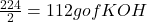1 mole of KOH = 39 + 16+ 1 = 56g

?mole of KOH = 112g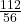moles of KOH = 2 moles/1liter of KOH

written as 2M KOH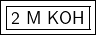Explanation:

Molarity is a measure of concentration in moles per liter.

### 1. Grams to Moles

The first step is to convert the amount of grams given to moles. The molar mass is used. This found on the Periodic Table and it’s the same value as the atomic mass, but the units are grams per mole.

We have 224 grams of KOH. Look up the molar masses for the individual elements.

• Potassium (K): 39.098 g/mol
• Oxygen (O): 15.999 g/mol
• Hydrogen (H): 1.008 g/mol

Since the compound’s formula has no subscripts, 1 formula unit has 1 atom of each element. We can simply add the molar masses together to find KOH’s molar mass.

• KOH: 39.098 + 15.999 + 1.008=56.105 g/mol

Use this number as a ratio.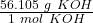Multiply by the value we are converting: 224 g KOH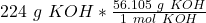Flip the ratio so the units of grams KOH cancel.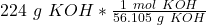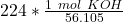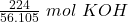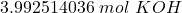### 2. Calculate Molarity

Remember molarity is moles per liter.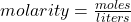We just calculated the moles and we know there are 2 liters of solution.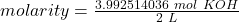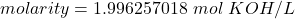### 3. Round and Convert Units

First, let’s round. The original values have 3 and 1 significant figures. We go with the lowest number: 1. For the number we found, that is the ones place.

• 1.996257018

The 9 in the tenths place tells us to round to 1 up to a 2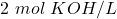Next, convert units. 1 mole per liter is equal to 1 molar or M.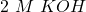The molarity of the solution is 2  M  KOH